## Info

Publication number
US20020057730A1
US20020057730A1 US09/921,917 US92191701A US2002057730A1 US 20020057730 A1 US20020057730 A1 US 20020057730A1 US 92191701 A US92191701 A US 92191701A US 2002057730 A1 US2002057730 A1 US 2002057730A1
Authority
US
United States
Prior art keywords
calculating
signal
zero rate
Prior art date
Legal status (The legal status is an assumption and is not a legal conclusion. Google has not performed a legal analysis and makes no representation as to the accuracy of the status listed.)
Abandoned
Application number
US09/921,917
Inventor
Jonas Karlsson
Jeonghoon Han
Current Assignee (The listed assignees may be inaccurate. Google has not performed a legal analysis and makes no representation or warranty as to the accuracy of the list.)
Telefonaktiebolaget LM Ericsson AB
Original Assignee
Telefonaktiebolaget LM Ericsson AB
Priority date (The priority date is an assumption and is not a legal conclusion. Google has not performed a legal analysis and makes no representation as to the accuracy of the date listed.)
Filing date
Publication date
Priority to US22303200P priority Critical
Application filed by Telefonaktiebolaget LM Ericsson AB filed Critical Telefonaktiebolaget LM Ericsson AB
Priority to US09/921,917 priority patent/US20020057730A1/en
Assigned to TELEFONAKTIEBOLAGET L M ERICSSON reassignment TELEFONAKTIEBOLAGET L M ERICSSON ASSIGNMENT OF ASSIGNORS INTEREST (SEE DOCUMENT FOR DETAILS). Assignors: HAN, JEONGHOON, KARLSSON, JONAS
Publication of US20020057730A1 publication Critical patent/US20020057730A1/en
Application status is Abandoned legal-status Critical

## Images

•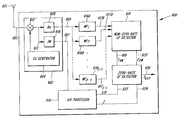•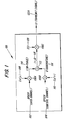••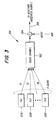•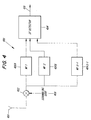•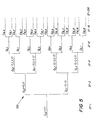•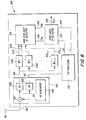••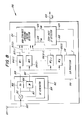•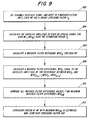•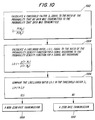•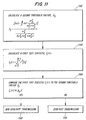•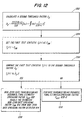•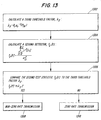•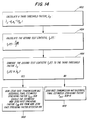•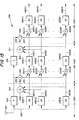•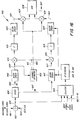••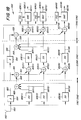•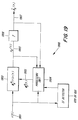•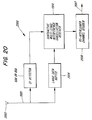•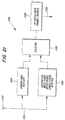•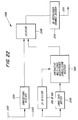## Classifications

• HELECTRICITY
• H04ELECTRIC COMMUNICATION TECHNIQUE
• H04BTRANSMISSION
• H04B1/00Details of transmission systems, not covered by a single one of groups H04B3/00 - H04B13/00; Details of transmission systems not characterised by the medium used for transmission
• H04B1/707Spread spectrum techniques using direct sequence modulation
• HELECTRICITY
• H04ELECTRIC COMMUNICATION TECHNIQUE
• H04BTRANSMISSION
• H04B2201/00Indexing scheme relating to details of transmission systems not covered by a single group of H04B3/00 - H04B13/00
• H04B2201/69Orthogonal indexing scheme relating to spread spectrum techniques in general
• H04B2201/707Orthogonal indexing scheme relating to spread spectrum techniques in general relating to direct sequence modulation
• H04B2201/70703Orthogonal indexing scheme relating to spread spectrum techniques in general relating to direct sequence modulation using multiple or variable rates
• H04B2201/70705Rate detection

## Abstract

A method for determining whether a zero rate or non-zero rate transmission has occurred, in a variable spreading factor CDMA system, which provides the solution to the problem of de-spreading received signals with incorrect spreading codes and reducing processing delays, is presented. The method utilizes information determined from the received signals, which include control and data channel information, to determine whether a zero rate or non-zero rate transmission has occurred, and generating the correct spreading factor based on that determination. The method is based in a spreading factor detector, which is subsequently able to be utilized in several types of multiple access interference cancellation receivers, which utilize interference cancellation techniques.

## Description

PRIORITY INFORMATION
• The present application claims priority under 35 U.S.C. §119(e) to U.S. Provisional application No. 60/223,032, filed on Aug. 4, 2000, the entire contents of which are herein expressly incorporated by reference.
• TECHNICAL FIELD OF THE INVENTION
• The present invention involves the field of telecommunication systems, and the use of Code Division Multiple Access (CDMA) communications techniques in cellular radio communication systems. In particular, the present invention relates to the reduction of processing delay in multiple access interference cancellation algorithms. 
• BACKGROUND
• In modern mobile communication, there are several multiple access schemes such as FDMA (Frequency division multiple access), TDMA (Time division multiple access), and CDMA. For the third generation mobile communication system defined by 3 rd Generation Partnership Project (3GPP), CDMA has been adopted because of its high frequency efficiency, low probability of intercept, and so forth, compared to the other multiple access systems, e.g., TDMA and FDMA.
• FIG. 1 illustrates a CDMA transmitter  100 based on Wideband CDMA system. The CDMA transmitter 100 combines a data channel (DPDCH: Dedicated Physical Data Channel) 102 and a control channel (DPCCH: Dedicated Physical Control Channel) 104, for transmission over an air interface. The DPDCH is used for transporting user data such as voice, data, etc. The data speed of the DPDCH varies (i.e. DPDCH is a variable data rate channel). The DPCCH is used for transporting control data.
• FIG. 2 illustrates the structure of the DPDCH and the DPCCH of the W-CDMA uplink. As shown in FIG. 2, the DPDCH and the DPCCH each comprise a plurality of slots of 0.667 ms in duration. Fifteen slots form one frame of 10 ms duration (15×0.667 ms=10 ms). One slot of the DPCCH comprises Pilot bits which are utilized for channel estimation, TFCI (Transport Format Combination Indicator) bits, FBI (Feedback Information) bits and TPC (Transmit Power Control) bits, TFCI bits provide the receiver with information about the DPDCH, i.e. spreading factor, coding rate, repetition pattern, etc. The information provided by the TFCI bits are spread across all the slots within one frame. 
• As shown in FIG. 1, the data of the DPDCH  102 is input to multiplier 106A, where it is spread by spreading code (channelization code) Cx(y) 108. The code length of the spreading code Cx(y) 108 varies in response to the data rate of the DPDCH 102. Generally, the code length of the spreading code is defined by the spreading factor (SF). For example, if the SF=4, the spreading code is 4 chips in length. FIG. 5 illustrates spreading codes used in W-CDMA systems. In a W-CDMA system uplink, the spreading factor can vary from SF=4 up to SF=256 in response to the data rate of the DPDCH 102. Although the spreading factor may vary every frame, it does not vary within the same frame.
• The control data of the (DPCCH)  104 is input to multiplier 106B. A second input of multiplier 106B is connected to spreading code Cu(v) 110 (in which the code length remains constant). The spread data channel and control channel output respectively from multipliers 106A and 106B are combined by adder 112. As illustrated in FIG. 1, the DPDCH is an In-phase signal and the DPDCH is a Quadrature-phase signal which are combined to form an I+jQ signal. The combined I+jQ signal is multiplied by scramble code SC1 120 by multiplier 106C. The scrambled combined data 122A from multiplier 106C is transformed into an RF signal by an RF circuit (not shown) and then is transmitted through an antenna (also not shown).
• FIG. 3 illustrates a radio channel model of a CDMA system  300. CDMA signals 122A-K from a plurality of CDMA transmitters 100A-K are propagated on the radio channel 302. Noise 306 is added to the transmitted RF signal at the receiver, as illustrated by adder 304. The combined signal 308 is a combination of noise 306 (atmospheric, intentional and unintentional interference, and receiver noise) and the transmitted CDMA signals 122A-K.
• It will be recognized that CDMA signals from other users (Tx 2-TxK) influence the CDMA signal of the user Tx1 as interference signals which degrade the performance of the data detection by the receiver of the information transmitted by transmitter Tx1 122A. This is an example of unintentional interference. Therefore, it is preferable to reduce interference signals.
• Various techniques for interference cancellation have been proposed in recent years. One of them is subtractive multi-stage interference cancellation. In subtractive multi-stage interference cancellation, by performing the de-spreading process and the symbol detection process to the received signal, data from each user is tentatively detected. The detected data is re-spread using spreading code of each user and the re-spread signals are subtracted from the received signal as replica signals of interference signals. The residual signal generated by the subtraction process is added to the re-spread signals of each user. Then, the de-spreading process, the symbol detection process and the re-spreading process are performed to the combined signals respectively. By repeating these processes, in a subtractive multi-stage interference cancellation, the influence of interference signals is reduced and performance of the data detection is improved. 
• Any of these interference cancellation techniques generates a processing delay due to the complexity of the process. In case of the above-described W-CDMA systems, since the code length of the spreading code used varies which spreading factor has been used must be detected before starting the interference cancellation. In W-CDMA systems, if the whole frame is received, it the spreading factor which has been used can be identified on the basis of TFCI bits. However, waiting to completely receive the whole data frame generates further process delay. Since some services, e.g., voice services, require short processing delay, in order to make it feasible to use interference cancellation techniques in commercial systems, the processing delay (other than the interference cancellation process) should be reduced as much as possible. 
• In the spreading factor detector  404, the mean-power is detected for each de-spread signal. The mean-power for each de-spread signal is shown as:
• {overscore (P)}x(x=1, . . . , X-1)
• The mean-power from each matched filter  406 is then compared to each other to determine the maximum mean-power. The spreading factor of the matched filter that corresponds to the maximum mean-power is selected, and the spreading factor detector 404 outputs the selected spreading factor as the spreading factor used for the received signal.
• As described above, in a W-CDMA system, user data is assigned to the I-channel and the control data is assigned to the Q-channel (see FIG. 1). When user data is not transmitted, the data channel is inactive but the control channel is active. This situation is referred to as “zero rate” transmission. When both channels are active, the transmission state is referred to as “non-zero rate” transmission. Further, the spreading factor at the time when a zero rate transmission has occurred is defined as spreading factor 0, or a “zero rate” spreading factor. 
• In the above-described conventional spreading factor detector  400, the situation of zero rate transmission has not been considered at all. Therefore, if applying the spreading factor detector 400 to a W-CDMA uplink, the accurate detection of the spreading factor could not be performed in all circumstances. That is, the spreading factor detector 400 can not accurately detect a zero rate spreading factor.
• SUMMARY OF THE INVENTION
• The invention involves a method for determining whether a zero rate transmission has occurred in a wide band code division multiple access communications system, comprising calculating a first threshold value, a likelihood ratio, and then comparing the first threshold value to the likelihood ratio. Based on the comparison, a non-zero rate transmission has occurred if the likelihood ratio is greater than or equal to the first threshold value, or determining that a zero rate transmission has occurred if the likelihood ratio is less than the first threshold value. 
• The invention involves a second method for determining whether a zero rate transmission has occurred in a wide band code division multiple access communications system, comprising calculating a second threshold value, λ 2, a first test statistic, T1(r) and then comparing the second threshold value to the first test statistic. Based on the comparison, a non-zero rate transmission has occurred if the first test statistic is greater than or equal to the second threshold value, or determining that a zero rate transmission has occurred if the first test statistic is less than the second threshold value.
• The invention involves a third method for determining whether a zero rate transmission has occurred in a wide band code division multiple access communications system, comprising calculating a third threshold value, λ 3, a second test statistic, T2(r), and then comparing the third threshold value to the second test statistic. Based on the comparison, a non-zero rate transmission has occurred if the second test statistic is greater than or equal to the third threshold value, or determining that a zero rate transmission has occurred if the second test statistic is less than the third threshold value.
• The invention also involves a spreading factor detector, for use in a wideband code division multiple access communications system, comprising a de-scrambler, with an input connected to a received baseband signal, and a real signal output, and an imaginary signal output, a SIR processor, with an input connected to the imaginary signal output, and a plurality of SIR processor outputs, a plurality of matched filters, each matched filter having an input connected to the real signal output, and a matched filter output. Additionally, the spreading factor detector comprises a non-zero rate spreading factor detector having a plurality of inputs connected to the plurality of matched filter outputs, and a plurality of non-zero rate spreading factor detector outputs, and a zero rate spreading factor detector having a plurality of inputs connected to the plurality of non-zero rate spreading factor detector outputs and the plurality of SIR processor outputs, and an estimated spreading factor output signal.
• BRIEF DESCRIPTION OF THE DRAWINGS
• The novel features believed characteristic of the invention are set forth in the appended claims. The invention itself, however, as well as other features and advantages thereof will be best understood by reference to the detailed description of the specific embodiments which follows, when read in conjunction with the accompanying drawings. 
• FIG. 1 illustrates a CDMA transmitter based on a Wideband CDMA (w-CDMA) system, which is defined by the 3 rd Generation Partnership Project (3GPP) as a 3rd generation cellular system;
• FIG. 2 illustrates the structure of the data channel and control channel in a w-CDMA uplink; 
• FIG. 3 illustrates a radio channel model of a CMDA system; 
• FIG. 4 illustrates a conventional spreading factor detector; 
• FIG. 5 illustrates a spreading code tree; 
• FIG. 6 illustrates a first spreading factor detector according to a preferred embodiment of the invention; 
• FIG. 7 illustrates a method for determining a non-zero rate spreading factor in the first spreading factor detector, according to an embodiment of the invention; 
• FIG. 8 illustrates a second spreading factor detector according to an embodiment of the invention; 
• FIG. 9 illustrates a method for determining a non-zero rate spreading factor in the second spreading factor detector, according to an embodiment of the invention; 
• FIG. 10 illustrates a method for determining whether a zero-rate or non-zero rate transmission has occurred according to an embodiment of the invention; 
• FIG. 11 illustrates a method for determining whether a zero rate or non-zero rate transmission has occurred according to an embodiment of the invention; 
• FIG. 12 illustrates a method for determining whether a zero or non-zero rate transmission has occurred according to an embodiment of the invention; 
• FIG. 13 illustrates a method for determining whether a zero rate or non-zero rate transmission has occurred according to an embodiment of the invention; 
• FIG. 14 illustrates a method for determining whether a zero rate or non-zero rate transmission has occurred according to an embodiment of the invention; 
• FIG. 15 illustrates a subtractive multi-stage interference cancellation receiver with a spreading factor detector of an embodiment of the invention; 
• FIG. 16 illustrates an interference cancellation unit with a spreading factor detector of an embodiment of the invention; 
• FIG. 17 illustrates input signals to an interference cancellation unit, in a subtractive multi-stage interference cancellation receiver; 
• FIG. 18 illustrates a modified multi-stage interference cancellation receiver with a spreading factor detector of an embodiment of the invention; 
• FIG. 19 illustrates an adaptive single user detector with a spreading factor detector of an embodiment of the invention; 
• FIG. 20 illustrates a large buffer interference cancellation receiver with a spreading factor detector of an embodiment of the invention; 
• FIG. 21 illustrates a parallel interference cancellation receiver with a spreading factor detector of an embodiment of the invention; and 
• FIG. 22 illustrates a buffer parallel interference cancellation receiver with a spreading factor detector of an embodiment of the invention.
• DETAILED DESCRIPTION OF THE INVENTION
• The various features of the invention will now be described with reference to the figures, in which like parts are identified with the same reference characters. 
• De-scrambled imaginary signal  607 is input to SIR processor 610. SIR processor 610 calculates the signal-to-interference ratio (SIR) based on the signal strength (S) and interference signal strength (I) (SIR=S/I). Methods of calculating the SIR are well known in the art, e.g., de-spreading the control channel, and calculating the signal strength and interference signal strength of the pilot bits which are placed within the control channel (i.e., DPCCH). SIR processor 610 outputs data channel SIR signal 622 and interference strength signal 624 to the zero rate spreading factor detector 626. Strictly speaking, data channel SIR signal 622 and interference strength signal 624 show the signal-to-interference ratio (SIR) and interference signal strength (I) of DPCCH. However, these values may be used as SIR and I of DPDCH. Of course, SIR and I of DPDCH may be calculated based on SIR and I of DPCCH according a predetermined method.
• The zero rate spreading factor detector  626 determines whether a zero rate or non-zero rate transmission has occurred and then outputs the final estimated spreading factor (Fest) 628. That is, the zero rate spreading factor detector 626 outputs the final estimated zero rate spreading factor 628 if a zero rate transmission has occurred, or the zero rate spreading factor detector 626 outputs the estimated non-zero rate spreading factor 618 if a non-zero rate transmission has occurred.
• FIG. 7 illustrates a method for determining a non-zero rate spreading factor in the first spreading factor detector, according to an embodiment of the invention. In step  702 the signal is received, de-scrambled, and then the real part of the de-scrambled signal is input to X-1 matched filters. Each matched filter de-spreads the received signal according to the spreading code based on the unique spreading factor Fx assigned to it.
• Steps  702-708 occur in the non zero rate spreading factor detector 614. In step 704, the mean-power P{overscore (x)} of each de-spread signal is calculated. In step 706, all the mean-power P{overscore (x)} are compared, to determine the maximum mean-power P{overscore (x)}m. In step 708, the spreading factor Fx of the matched filter that corresponds to the maximum mean-power is selected as the estimated non-zero rate spreading factor 618.
• Operation of the zero rate spreading factor detector  626 is described in the method illustrated in FIGS. 10-14. The zero rate spreading factor detector 626 is used also in the second spreading factor detector 800 illustrated in FIG. 8 (zero rate spreading factor detector 826).
• FIG. 8 illustrates a second spreading factor detector according to an embodiment of the invention. The difference between the first spreading factor detector  600 and the second spreading factor detector 800 is an additional matched filter in the second spreading factor detector 800, and the method for estimating the non-zero rate spreading factor.
• In FIG. 8, it is assumed that the spreading factors and associated spreading codes used by the CDMA transmitter are known to the CDMA receiver, i.e., there is a finite known set of spreading factors and codes. Additionally, the possible spreading codes are in the same code branch. In FIG. 8, de-scrambled imaginary signal  807 (obtained by de-scrambler 802) is input to SIR processor 810, and then the data channel SIR signal 822 and interference strength signal 824 are calculated, and input to zero-rate spreading factor detector 826.
• The zero rate spreading factor detector  826 determines whether a zero rate or non-zero rate transmission has occurred and then outputs the final estimated spreading factor 828. That is, the zero rate spreading factor detector 826 outputs the zero rate spreading factor if a zero rate transmission has occurred, or the zero rate spreading factor detector 826 outputs the estimated non-zero rate spreading factor 818 if a non-zero rate transmission has occurred.
• FIG. 9 illustrates a method for determining a non-zero rate spreading factor in the second spreading factor detector, according to an embodiment of the invention. The method of FIG. 9 is used in conjunction with the second spreading factor detector  800 illustrated in FIG. 8. In step 902 the signal is received, de-scrambled, and input to X matched filters. Each matched filter de-spreads the received signal according to its assigned spreading code.
• Steps  904-912 occur in the non-zero rate spreading factor detector 814. In step 904, the absolute amplitude of each de-spread signal is calculated. The absolute amplitude of each de-spread signal is calculated by summing, individually, the outputs from X matched filters over a period of time equal to the duration of the spreading code (estimation period, Te) and taking the absolute value of the result. The amplitude signal of the Xth matched filter can be represented as MFAX. The absolute amplitude signal from the Xth matched filter is therefore represented as |MFAX|.
• In step  906 a matched filter integrand is calculated for each |MFAX|. The Xth matched filter integrand is shown as MFAIX.
• The matched filter integrand is defined as:  $MFAI X = ∫ 0 T e   MFA X    t$
• where T e is the estimation period.
• In step  908, the matched filter difference, MFDX is calculated. The matched filter difference is defined as:
• MFDX=|MFAIX−MFAIX+1| (X=1, 2, . . . , X−1)
• In step  910, all the matched filter difference values are compared. The maximum matched filter difference is found (represented as MFDXM). In step 912, the spreading factor Fx of the matched filter that corresponds to the maximum matched filter difference MFDXM is selected as the estimated non-zero rate spreading factor 818.
• Mathematically, the operation of steps  902-910 can be shown as follows:
• Z X(t) is the output signal from the X-th matched filter. The absolute amplitude of ZX(t) is |ZX(t)| and is equivalent to |MFAX| calculated in step 904. Then, $v x = ∫ 0 T e   Z x  ( t )    t$
• where v x is the matched filter integrand MFAIX (step 906). A row vector v={v1, v2, . . . , vX} can be written, so that a difference vector w={w1, w2, . . . , wX} can be determined, where wX is defined as:
• w X =|v x −v x+1|, (X=1, 2, . . . , X−1)
• This is equivalent to MFD X according to step 908. Table 1 shows the calculations determining wX when the number of matched filters X=8.  TABLE 1 X MFDX |MFAIX - MFAIX+1| 1 MFD1 |MFAI1 - MFAI2| 2 MFD2 |MFAI2 - MFAI3| 3 MFD3 |MFAI3 - MFAI4| 4 MFD4 |MFAI4 - MFAI5| 5 MFD5 |MFAI5 - MFAI6| 6 MFD6 |MFAI6 - MFAI7| 7 MFD7 |MEAI7 - MFAI8|
• The spreading factor of the matched filter associated with the maximum vector w is the estimated non-zero rate spreading factor  818. For example, if w4 is the maximum w vector, then spreading factor of 4th matched filter is the estimated non-zero rate spreading factor 818.
• The method of FIG. 7, previously described in relation to the first spreading factor detector  600 illustrated in FIG. 6, may also be used in the second spreading factor detector 800 illustrated in FIG. 8. However, in order for the method illustrated in FIG. 7 to be used in the second spreading factor detector 800, the output of the last matched filter 816(X) must be terminated; i.e., not connected to the non-zero rate spreading factor detector 814.
• FIG. 10 illustrates a method for determining whether a zero-rate or non-zero rate transmission has occurred according to an embodiment of the invention. Several assumptions regarding the input signals are necessary in order to derive the method of FIG. 10. It is assumed the signal, s, is a zero mean, Gaussian, random process with a variance σ s 2. Noise, no, is AWGN (Additive White Gaussian Noise) with variance $σ 0 2 .$
• . That is, r˜N(0,σ o 2I) under H0 and r˜N(0,(σs 2o 2)I) under H1, where I is a unity matrix and 0 is an all zero matrix. The notation N(μ,σ2) denotes a Gaussian probability density function (pdf) with mean μ and variance σ2.
• The steps for determining whether a zero rate or non-zero rate transmission has occurred, take place in the zero rate spreading factor detectors  626 and 826 of FIGS. 6 and 8. The problem to be solved is to determine which transmission has occurred:
• H 0 r[n]=n 0 [n]: No data transmitted, i.e., zero rate transmission
• H 1 r[n]=s[n]+n 0 [n]: Data transmission, i.e., non-zero rate transmission.
• In these equations, n=0, 1, 2, . . . N−1. N is the number of bits for T e the estimation period. H0 is the “noise only” scenario, (spreading factor equal to 0 is used) and H1 is the “signal present” scenario (the non-zero rate spreading factor is used); and r[n] signifies the received signal, i.e., the n-th received bit.
• In step  1002, a Threshold Factor λ is determined, using the following equation: $λ = P  ( H 0 ) P  ( H 1 )$
• where P(H 0) is the probability that no data was transmitted, or “noise only.” P(H1) is the probability that data was transmitted, i.e. “signal present.” In general, these probabilities are not known. In lieu of knowing the probabilities, there are two alternate methods for calculating the Threshold Factor λ: the first is that the probabilities can either be set to fixed values (i.e., the probability of no data transmission equals a first fixed value, and the probability of data transmission equals a second fixed value), or alternatively, the probabilities can be adaptively set using information of the past received signal and a traffic model for the used service. In the latter case, the “fixed” values would be empirically determined over time.
• In step  1004, a Likelihood Ratio, L(r) is determined: $L  ( r ) = p  ( r ; H 1 ) p  ( r ; H 0 )$
• The numerator in the equation to determine L(r) is the value determined by the probability density function for a signal occurring, and the denominator is the value of the probability density function for a signal not occurring. 
• In step  1006, the Likelihood Ratio L(r) is compared to the Threshold Factor λ:
• If L(r)≧λ (step  1008) then the transmission is a non-zero rate transmission (i.e. signal present) (“Yes” path out of decision step 1006).
• If L(r)<λ (step  1010) then the transmission is a zero rate transmission (i.e. no signal present) (“No” path out of decision step 1006).
• FIG. 11 illustrates a method for determining whether a zero rate or non-zero rate transmission has occurred according to an embodiment of the invention. The method illustrated in FIG. 11 is based on a statistical analysis of transmitted signals. Because it was assumed that the input signals are a Gaussian random process, the probability density functions in the Likelihood Ratio L(r) can be replaced by the probability density functions of a Gaussian random variable. Then, the Likelihood Ratio L(r) can be written as:  $L  ( r ) = 1 [ 2  π  ( σ s 2 + σ o 2 ) ] N / 2  exp [ - 1 2  ( σ s 2 + σ o 2 )  ∑ n = 0 N - 1  r 2  [ n ] 1 [ 2  π   σ o 2 ] N / 2  exp [ - 1 2  σ o 2  ∑ n = 0 N - 1  r 2  [ n ]$
• Then we take logarithm with respect to L(r), so that the log-Likelihood Ratio l(r) is:  $l  ( r ) = N 2  ln  ( σ o 2 σ s 2 + σ o 2 ) + 1 2  [ σ s 2 σ o 2  ( σ s 2 + σ o 2 ) ]  ∑ n = 0 N - 1  r 2  [ n ]$
• We can rewrite the equation in step  1006 for Likelihood Ratio L(r) and Threshold Factor λ, by taking the logarithm of both sides (recall that step 1006 was written as: L(r)≧λ):
• l(r)≧ln(λ)
• By rearranging the above equations (for l(r)), we have:  $∑ n = 0 N - 1  r 2  [ n ] ≥ λ 2$
• Here, we define a First Test Statistic T 1(r) as: $T 1  ( r ) = ∑ n = 0 N - 1  r 2  [ n ]$
• We define a Second Threshold Factor λ 2 as: $λ 2 = [ ln   λ - N 2  ln  ( σ o 2 σ s 2 + σ o 2 ) ] / [ σ s 2 2  σ o 2  ( σ s 2 + σ o 2 ) ]$
• In step  1102 the method calculates the Second Threshold Factor λ2: $λ 2 = [ ln   λ - N 2  ln  ( σ o 2 σ s 2 + σ o 2 ) ] / [ σ s 2 2  σ o 2  ( σ s 2 + σ o 2 ) ]$
• As before, the value of the Threshold Factor λ is based on empirical measurements. 
• In step  1104, the First Test Statistic T1(r) is calculated: $T 1  ( r ) = ∑ n = 0 N - 1  r 2  [ n ]$
• where r is the received signal. 
• In step  1106, the First Test Statistic T1(r) is compared to the Second Threshold Factor λ2:
• If T 1(r)≧λ2 (step 1108) then the transmission is a non-zero rate transmission, i.e., signal present (“Yes” path out of decision step 1106).
• If T 1(r)<λ2 (step 1110) then the transmission is a zero rate transmission, i.e., no signal present (“No” path out of decision step 1106).
• FIG. 12 illustrates a method for determining whether a zero or non-zero rate transmission has occurred according to an embodiment of the invention. The method illustrated in FIG. 12, for determining whether a non-zero rate or zero rate transmission has occurred, is used in conjunction with the spreading factor detectors  600 and 800 of FIGS. 6 and 8. The method illustrated in FIG. 12 is itself based on the method illustrated in FIG. 11. The method illustrated in FIG. 12 presupposes that a determination of the estimated non-zero rate spreading factor 818 (or 618) has already been determined. In essence, FIG. 12 begins where the methods of FIGS. 7 and 9 ends, i.e., now, with these additional steps, it is possible to determine whether a zero rate or non-zero rate transmission has occurred. The steps of the method illustrated in FIG. 12 occur in the zero rate spreading factor detector 626 (or 826).
• There are three signals used in the method of FIG. 12: These are the estimated non-zero spreading factor  618 (or 818), data channel signal energy 620 (or 820) (EXM), and the data channel SIR signal 622 (or 822). The method of FIG. 12 does not need the interference strength signal 624 (or 824) from SIR processor 610 (or 810). Each input can be represented as follows: $SIR  ( 822 ) = S I ;$
• the calculated signal-to-interference 
• ratio for DPDCH  102, calculated by SIR processor; $E XM = ∫ 0 Te  p XM$
• is the calculated data channel signal energy from the matched filter having the estimated spreading factor; and 
• F XM is the estimated non-zero rate spreading factor from the non-zero rate spreading factor detector.
• In step  1202, data channel SIR signal 622 (or 822), interference strength signal 624 (or 824), and the First Threshold Factor λ are used to calculate the Second Threshold Factor λ2: $λ 2 = 2  I  ( SIR + 1 ) SIR  [ ln   λ - N 2  ln  ( 1 SIR + 1 ) ]$
• In step  1204, the data channel signal energy EXM 620 (or 820) is set to the First Test Statistic T1(r), which is derived from FIG. 11:
• T 1(r)=E XM
• In step  1206, the Second Threshold Factor λ2 is compared to the First Test Statistic T1(r), (i.e., the data channel signal energy EXM 620 (or 820)) from the matched filter 616 (or 816) having the estimated non-zero rate spreading factor 618 (or 818):
• If T 1(r)≧λ2 (step 1208), then a non-zero rate transmission has occurred (“Yes” path out of decision step 1206). The estimated non-zero spreading factor FXM from the non-zero rate detector is the final estimated spreading factor Fest.
• If T 1(r)≧λ2 (step 1210), then a zero rate transmission has occurred (“No” path out of decision step 1206). In this instance the final estimated spreading factor Fest is zero.
• FIG. 13 illustrates a method for determining whether a zero rate or non-zero rate transmission has occurred according to an embodiment of the invention. The second statistical analysis method for determining whether a zero rate or non-zero rate transmission has occurred is based on the chi-squared probability density function (pdf) of r with v degree of freedom, where:  $r = ∑ i = 0 v  r i 2$
• The right-tail probability of R v 2 random variable is defined for even v as: $Q R v 2 = exp   ( - 1 2  r )  ∑ k - 0 v / 2 - 1  ( r / 2 ) 2 k !$
• The probability of deciding H 1 (non-zero rate transmission), when H0 (zero-rate transmission) is true, is referred to as probability of false alarm PFA:
• P FA =P{T 1(r)>λ2 ;H 0} $P FA = P  { T 1  ( r ) > λ 2 ; H 0 } = P  { T 1  ( r ) / σ 0 2 > λ 2 / σ 0 2 ; H 0 } = Q R v 2  ( r / σ 0 2 )$  =Q R v 2 (r/σ 0 2)
• The probability o f a false alarm is the value of the chi-squared probability density function given that T 1(r) is larger than λ2, when a zero-rate transmission has occurred. In other words, its the probability a mistake is made in ascertaining when a non-zero rate transmission has occurred, when in actuality a zero-rate transmission has occurred. Ideally, false alarm probabilities should be as small as possible, and even more ideally, negligible.
• A Second Test Statistic T 2(r), is defined as follows: $T 2  ( r ) = T 1  ( r ) σ 0 2 > Q R v 2 - 1  ( P FA )$
• A Third Threshold Factor λ 3 is defined as: $λ 3 = Q R v 2 - 1  ( P FA ) ;$
• where Q R 2 −1 is the inverse of the right-tail probability for a Rv 2 random variable.
• In step  1302 the method calculates the Third Threshold Factor λ3: $λ 3 = Q R v 2 - 1  ( P FA )$
• where the value of P FA is decided on empirical measurements or is adaptively set on the basis of the past received signal and other conditions.
• In step  1304, the Second Test Statistic, T2(r) is calculated: $T 2  ( r ) = ∑ n = 0 N - 1  r 2  [ n ] / σ 0 2$
• In step  1306, the Second Test Statistic T2(r) is compared to the Third Threshold Factor λ3:
• If T 2(r)≧λ3 (step 1308) then the transmission is a non-zero rate transmission, i.e., signal present (“Yes” path out of decision step 1306).
• If T 2(r)<λ3 (step 1310) then the transmission is a zero rate transmission, i.e., no signal present (“No” path out of decision step 1306).
• FIG. 14 illustrates a method for determining whether a zero rate or non-zero rate transmission has occurred according to an embodiment of the invention. The method illustrated in FIG. 14, is used in conjunction with the spreading factor detectors  600 and 800 of FIGS. 6 and 8. The method illustrated in FIG. 14 is itself based on the method illustrated in FIG. 13. The method illustrated in FIG. 14 presupposes that a determination of the estimated non-zero rate spreading factor 618 (or 818) has already been determined. In essence, FIG. 14 (as was the case regarding FIG. 12), begins where the method of FIG. 7 or 9 ends, i.e., now, with these additional steps, it is possible to determine whether a zero rate or non-zero rate transmission has occurred. The steps of the method illustrated in FIG. 14 occur in the zero rate spreading factor detector 626 (or 826).
• There are three signals used in the method of FIG. 14. These are the estimated non-zero rate spreading factor  618 (or 818), the data channel signal energy 620 (or 820), and interference strength signal 624 (or 824). The method does not utilize the data channel SIR signal 622 (or 822) from SIR processor 610 (or 810).
• The data channel signal energy (E XM) 620 (or 820), is determined by integrating the mean power of the signal generated by the matched filter 616 (or 816) that produced the estimated non-zero rate spreading factor (FXM) 620 (or 820), over a period of time equal to the estimation period, Te: $E XM = ∫ 0 Te  p XM$
• In step  1402, interference strength signal (I) 624 (or 824) and the Threshold Factor λ are used to calculate the Third Threshold Factor λ3: $λ 3 = Q R v 2 - 1  ( P FA )$
• In step  1404, the Second Test Statistic, T2(r) is calculated: $T 2  ( r ) = E XM I$
• The Second Test Statistic T 2(r) is defined as the ratio of the signal energy of the matched filter producing the estimated non-zero rate spreading factor FXM 620 (or 820), to the interference strength signal I 624 (or 824).
• In step  1406, the Third Threshold Factor λ3 is compared to the second test statistic T2(r).
• If T 2(r)≧λ3 (step 1408) then a non-zero rate transmission has occurred (“Yes” path out of decision step 1406). The estimated non-zero rate spreading factor FXM from the non-zero rate spreading factor detector is the final estimated spreading factor Fest.
• If T 2(r)<λ3 (step 1410) then a zero rate transmission has occurred (“No” path out of decision step 1408). In this instance the final estimated spreading factor Fest is zero.
• The first sub-type is a subtractive multi-stage IC receiver, discussed with regards to FIGS.  15-18; a second sub-type is an adaptive single user detector, discussed with regards to FIG. 19; a third sub-type is a large buffer IC receiver, discussed with regards to FIG. 20; and the last, and fourth sub-type is a parallel interference cancellation receiver, discussed with regard to FIGS. 21-22. Each will be discussed in turn.
• FIG. 16 illustrates an interference cancellation unit with a spreading factor detector of an embodiment of the invention. FIG. 16 illustrates ICUs  1506, 1514 and 1522, as shown in FIG. 15. In ICU 1600, an input signal 1619 from a first adder 1602 is input to a de-scrambler 1604 and a selector 1606. In case of the first stage, the input signal 1619 is the baseband signal 1601, and in case of the second and following stage, the input signal 1619 is the signal generated by adding the residual signal to the output signal (replica signal) 1603 from the prior stage.
• The selector  1606 has other input signals 1607(1)-1607(N). These signals are the output signals of ICUs of other stages. Further, the selector 1606 has a buffer to store input signals, and selects one or a plurality of input signals from the stored signals and then outputs them to the spreading factor detector 800 (or 600). The spreading factor detector 800 (or 600) determines a final estimated spreading factor 628 (or 828) used in the data channel (DPDCH) and then outputs it to a de-spreader 1612 and a re-spreader 1622.
• De-scrambler  1604 has a de-scramble code generator, and de-scrambles input signal 1619 according to the de-scramble code output from the de-scramble code generator. In de-scrambler 1604, a real component (Re) 1605 and an imaginary component (Im) 1607 of the de-scrambled signal are extracted. The real component 1605 is output to de-spreader 1608 and the imaginary component 1607 is output to de-spreader 1612.
• The first de-spreader  1608 is a de-spreader for the control channel (DPCCH), and de-spreads the input signal 1619 according to a spreading code of the control channel, and then outputs the de-spread input signal 1609 to a multiplier 1614 and a channel estimator 1610.
• The channel estimator  1610 estimates a channel variation on the basis of the pilot bits of the control channel. The channel estimator 1610 has two outputs: the first is a complex conjugate estimated channel factor 1611, and the second is a channel factor 1613. The complex conjugate estimated channel factor 1611 is input to a first multiplier 1614 and a second multiplier 1615. The channel factor 1613 is input to a third multiplier 1624 and a fourth multiplier 1625.
• The first multiplier  1614, by multiplying the de-spread real signal 1609 with a conjugate value of the channel factor (complex conjugate estimated channel factor 1611), reduces the influence of the channel variation. A first detector 1616 tentatively detects symbols of the control channel from the output signal from the first multiplier 1614. A first re-spreader 1620, by re-spreading the detected symbols according to the spreading code for the control channel, generates the re-spread signal.
• A second de-spreader  1612 for the data channel (DPDCH) also has a spreading code generator, and generates a spreading code corresponding to the final estimated spreading factor 628 (or 828) determined by the spreading factor detector 800 (or 600). Then, the second de-spreader 1612 de-spreads the Im signal 16-7 according to the spreading code and outputs the de-spread Im signal 1617 to a second multiplier 1615.
• The second multiplier  1615, in the same manner as the first multiplier 1614, multiplies the de-spread IM signal 1617 with a conjugate value of the channel factor (complex conjugate estimated channel factor 1611) which reduces the influence of the channel variation. A second detector 1618 tentatively detects symbols of the control channel from the output signal from the second multiplier 1615.
• A second re-spreader  1622 also has a spreading code generator, and generates a spreading code corresponding to the final estimated spreading factor 628 (or 828) determined by the spreading factor detector 800 or (600). Then, the second re-spreader 1622 re-spreads the detected symbols and outputs the re-spread signal to fourth multiplier 1625.
• The third and fourth multipliers  1624 and 1625 multiplies the input signal with re-spread Re signal 1621 and re-spread Im signal 1623, respectively, the channel factor 1613 respectively, which generates a signal which incorporates the influence of channel variation. Scrambler 1626 combines the signals output from multipliers 1624 and 1625, and then generates scrambled ICU output signal 1627 by multiplying the combined third and fourth multiplier output signals by a scrambling code. Thus, ICU 1600, by performing a de-spreading and re-spreading process, generates a replica signal of each user, which is regarded by other users as an interference signal.
• As described above, the subtractive multi-stage interference cancellation receiver  1500 needs to know the final estimated spreading factor 628 (or 828) before starting the interference cancellation process. By using the spreading factor detector 800 (or 600), the receiver 1500 can utilize the spreading factor. However, because there are multiple stages in the subtractive multi-stage interference cancellation receiver 1500, implementation of the spreading factor detector 800 (or 600) can be more sophisticated.
• FIG. 17 illustrates input signals to an interference cancellation unit, in a subtractive multi-stage interference cancellation receiver. The signal  1702 shows the input signal to the first stage, the signal 1704 shows the input signal to the second stage, and signal 1706 shows the input signal to the third stage. Notation a, b and c means the first stage input signal, the second stage input signal and the third stage input signal, respectively. Further, the subscript number of notation 1, 2 and 3 means a slot number.
• In the subtractive multi-stage interference cancellation receiver  1500, the input signals will be delayed compared with each other, because of the processing delay in each stage. For example, in FIG. 17, the time difference between the first stage and the second stage is D1 (which is equal to approximately one slot), and the time difference between the first stage and the third stage is D2 (which is equal to approximately two slots). This means, for example, the signal of the slot 1 (which is shown in FIG. 17 by notation a1, b1, and c1) arrives at the second stage with the time delay D1, and arrives at the third stage with the time delay D2.
• The difference between the input signals  1702, 1704, 1706 is that the input signal 1702 is the signal upon which that the interference cancellation process has not yet been performed, the input signal 1704 is the signal that the interference cancellation process has been performed one time, and the input signal 1706 is the signal that the interference cancellation process has been performed two times. For example, signal 1702 could be the input to ICU 1506(1) of FIG. 15. Signal 1704 could be the input to ICU 1514(1), and signal 1706 the input to ICU 1522(1).
• The accuracy of the final estimated spreading factor  628 (or 828) will vary depending on which information is utilized. For example, when estimating the spreading factor for a certain slot, if using not only the information from the slot but also the information from the previous slots, a more accurate final estimated spreading factor 628 (or 828) will be obtained, because the amount of the data available to estimate the spreading factor increases. Further, greater accuracy will be obtained if still more information from other stages (post interference cancellation) is used because of the interference cancellation process.
• Thus, various systems and methods for estimating the spreading factor in a subtractive multi-stage interference cancellation receiver are available depending on which information is used. The systems and methods for providing for enhanced spreading factor detection are described more fully below. 
• Because it is important to use information from the previous slots, buffering the previous slots is necessary. Further, in order to use the information from other stages, the signal from other stages must be input to the spreading factor detector. For that reason, as shown in FIG. 16, a selector  1606 having a buffer is provided before the spreading factor detector 800 (or 600), so that the information from previous slots and other stages can be utilized and any information needed among the stored information can be selected and input to the spreading factor detector.
• In the descriptions below, the following notations are used: 
• a x=the first stage, slot x
• b x=the second stage, slot x
• c x=the third stage, slot x
• SF(a x)=Spreading Factor for slot x of the first stage
• SF(b x)=Spreading Factor for slot x of the second stage
• SF(c x)=Spreading Factor for slot x of the third stage
• Σ means that different slots are taken into account, but not that the spreading factors are added together. 
• The first method for determining the spreading factor for each slot of each stage, which is used when the stages are considered independently, is based on a cumulative determination based on previous slots. That is;  $SF  ( a x ) = ∑ i = 1 x  a i   for   1 ≤ x ≤ N$ $SF  ( b x ) = ∑ i = 1 x  b i   for   1 ≤ x ≤ N$ $SF  ( c x ) = ∑ i = 1 x  c i   for   1 ≤ x ≤ N$
• As an example, to estimate the spreading factor for slot “a 2”, slot “a1” and slot “a2” are used by the spreading factor detector. To estimate the spreading factor for slot “a3”, slot “a1”, “a2” and “a3” are used by the spreading factor detector.
• This process then repeats itself for all the slots in the first stage, and is the same regardless of the stage, except, of course, that for different stages, the respective slots would be used. 
• The second method for providing enhanced spreading factor detector in a multi-stage receiver, when the stages are considered completely dependent, is to use information from all the stages to establish the spreading factor. That is;  $SF  ( a x ) = ∑ i = 1 x  a i x = 1 ∑ i = 1 x  a i + ∑ i = 1 x - 1  b i x = 2 ∑ i = 1 x  a i + ∑ i = 1 x - 1  b i + ∑ i = 1 x - 2  c i 3 ≤ x ≤ N$ $SF  ( b x ) = ∑ i = 1 x + 1  a i + ∑ i = 1 x  b i x = 1 ∑ i = 1 x + 1  a i + ∑ i = 1 x  b i + ∑ i = 1 x - 1  c i 2 ≤ x ≤ N - 1 ∑ i = 1 x  a i + ∑ i = 1 x  b i + ∑ i = 1 x - 1  c i x = N$ $SF  ( c x ) = ∑ i = 1 x + 2  a i + ∑ i = 1 x + 1  b i + ∑ i = 1 x  c i 1 ≤ x ≤ N - 2 ∑ i = 1 x + 1  a i + ∑ i = 1 x + 1  b i + ∑ i = 1 x  c i x = N - 1 ∑ i = 1 x  a i + ∑ i = 1 x  b i + ∑ i = 1 x  c i x = N$
• As an example, to estimate the spreading factor for slot “a 1”, slot “a1” is used by the spreading factor detector.
• To estimate the spreading factor for slot “a 2” and “b1”, slot “a1”, “a2”, and “b2” are used by the spreading factor detector.
• To estimate the spreading factor for slot “a 3”, “a2” and “c1”, slot “a1”, “a2”, “a3”, “b1”, “b2” and “c1” are used by the spreading factor detector. This process is then repeated for all slots in the frame.
• The third method of providing enhanced spreading factor detection in a multi-stage receiver, when the stages are considered quasi-dependently, uses less than all the information from all stages. This is an intermediate solution, between the first and the second in complexity and precision, and reduces the complexity of calculating the spreading factor. The spreading factors are determined according to the following;  $SF  ( a x ) = ∑ i = 1 x  a i x = 1 ∑ i = 1 x  a i + ∑ i = 1 x - 1  b i 2 ≤ x ≤ N$ $SF  ( b x ) = ∑ i = 1 x + 1  a i + ∑ i = 1 x  b i 1 ≤ x ≤ N - 1 ∑ i = 1 x  a i + ∑ i = 1 x  b i x = N$ $SF  ( c x ) = ∑ i = 1 x + 2  a i + ∑ i = 1 x + 1  b i 1 ≤ x ≤ N - 2 ∑ i = 1 x + 1  a i + ∑ i = 1 x + 1  b i 1 ≤ x ≤ N - 1 ∑ i = 1 x  a i + ∑ i = 1 x  b i x = N$
• As an example, to estimate the spreading factor for slot “a 1”, slot “a1”, is used by the spreading factor detector.
• To estimate the spreading factor for slots “a 2” and “b1”, slot “a1”, “a2” and “b1” are used by the spreading factor detector.
• To estimate the spreading factor for slots “a 3”, “b2” and “c1”, slot “a1”, “a2”, “a3”, “b1” and “b1” are used by the spreading factor detector. Note that “c1” is not used here. This process then repeats itself.
• The fourth method of providing enhanced spreading factor detection, when the stages are considered dependent of each other, but not utilizing the current slot, uses information from only previous slots to establish the spreading factor. This calculation is much less complex than the first second or third method, yet produces fairly good performance. 
• If the processing time must be kept to a minimum, it will not be acceptable to wait until the current slot is completely input. However, since this fourth method does not use the current slot, it is able to reduce the delay corresponding to one slot. 
• In the fourth method, the spreading factor are determined according to the following;  $No   blind    SF   detection  x = 1$ $S   F  ( a x ) =  ∑ i = 1 x - 1  a i + ∑ i = 1 x - 1  b i x = 2 ∑ i = 1 x - 1  a i + ∑ i = 1 x - 1  b i + ∑ i = 1 x - 2  c i 3 ≤ x ≤ N$
• In this method, with regard to the slot “a 1” the spreading factor estimation process is not performed by the spreading factor detector. Instead, for example, the shortest spreading factor of the possible spreading factors is selected as a spreading factor for the slot “a1” and then it is output from the spreading factor detector.
• To estimate the spreading factor for slots “a 2”, slot “a1” and “b1” is used by the spreading factor detector.
• To estimate the spreading factor for slots “a 3”, slot “a1”, “a2”, “b1”, “b2” and “c1” is used by the spreading factor detector. This process then repeats itself for all slots.
• For other stages, the spreading factor SF(a x) is used.
• The amount of data used for the spreading factor estimation depends on the delay between the stages and other conditions. However, the above-described methods can estimate the spreading factor without waiting until the whole frame is received. Therefore, the above-described methods will substantially reduce the processing delay in a variable spreading factor CDMA system and make it feasible to implement interference cancellation receivers, which can increase the capacity, the range and/or lower the output power of the mobile terminals, in commercial systems. 
• FIG. 18 illustrates a modified multi-stage interference cancellation receiver with a spreading factor detector of an embodiment of the invention. A W-CDMA receiver configuration exists in which it is acceptable to wait until an entire frame has been received prior to determining the spreading factor. In this W-CDMA receiver configuration, determination of the final estimated spreading factor  628 (or 828) is made on the basis of the TFCI bits of the control channel. FIG. 18 illustrates a modified multi-stage interference cancellation receiver 1800 which institutes determination of the non-zero rate spreading factor in a TFCI detector.
• The difference between the modified multi-stage interference cancellation receiver of FIG. 18 and the unmodified version (FIG. 15) is the replacement in the third stage of the ICUs with RAKE receivers  1826, and the addition of TFCI detectors 1824, one for each RAKE receiver 1826. The input of each TFCI detector 1824 is connected directly to the baseband signal 1803 and the output of the TFCI detector 1824 is connected to a second input of the aforementioned RAKE receiver 1826.
• Each TFCI detector  1824 determines a spreading factor on the basis of the TFCI bits of the control channel of each user. That is, each TFCI detector 1824 receives an entire frame and determines the spreading factor on the basis of the TFCI bits distributed within the frame. The spreading factor determined by each
• SF(c x)=SF TFCIX 1≦x≦N
• As an example, to estimate the spreading factor for slot “a 1”, slot “a1” is used by the spreading factor.
• To estimate the spreading factor for slot “a 2”, slot “b2” is used by the spreading factor detector.
• To estimate the spreading factor for slot “a 3”, slot “b1” and “b2” are used by the spreading factor detector.
• To estimate the spreading factor for slot “b 1” slot “b1” is used by the spreading factor detector.
• To estimate the spreading factor for slot “b 2”, slot “b1” and “b2” are used by the spreading factor detector.
• To estimate the spreading factor for slot “b 3”, slot “b1”, “b2” and “b3” are used by the spreading factor detector. This process is then repeated for all slots in the frame.
• As described, the method for determining a non-zero rate spreading factor according to the modified multistage interference cancellation receiver  1800 requires a delay of one frame period (10 MS). Although this is a significant delay, when accurate symbol detection is desired this method is preferred. However, since the interference cancellation process has already been completed when the non-zero rate spreading factor is detected based on the TFCI bits, the receiver 1800 is able to start the final symbol detection process immediately after the spreading factor has been detected. Therefore, compared with starting the TFCI detector 1824(1)-(N) is output to its associated RAKE receiver 1826(1)-(N), respectively.
• Each ICU  1806, 1816 of the first and second stage (respectively) has the spreading factor detector 800 (or 600) (as shown in FIG. 16). ICUs 1806 and 1816 perform the interference cancellation process using the final estimated spreading factor 628 (or 828) determined by the spreading factor detector 800 (or 600). The received signal of each user is input to the rake receivers 1826(1)-(N).
• Each RAKE receiver  1826 contains a buffer of sufficient size to store at least one frame of the baseband W-CDMA signal 1803. TFCI detector 1824 determines the spreading factor of the control channel, and then outputs it to the RAKE receiver 1826. The RAKE receiver 1826, having stored an entire frame of received signal, then uses the TFCI generated spreading factor to perform a de-spreading process on the stored received signal. The detected symbols are then output as modified multi-stage interference cancellation receiver output signals 1827.
• The spreading factor detector  800 (or 600) within each ICU estimate the final estimated spreading factor 628 (or 828) according to the following method: $S   F  ( a x ) =  ∑ i = 1 x  a i x = 1 ∑ i = 1 x - 1  b i 2 ≤ x ≤ N$ $S   F  ( b x ) =  ∑ i = 1 x  b x 1 ≤ x ≤ N$
• interference cancellation process after the spreading factor is detected based on the TFCI bits, this method can reduce the overall processing delay. 
• FIG. 19 illustrates an adaptive single user detector with a spreading factor detector of an embodiment of the invention. Single user detectors are well known in the art, and are considered a species of interference cancellation techniques. Interference cancellation techniques are proposed as one of the methods to reduce the cross-correlation from other users. There are at least two well known interference cancellation techniques. The first is a multi-user detector that demodulates not only the desired signal of the intended channel, but also the signals of other simultaneous users received at the receiver, using the spreading code information of the other users. The second is a single user detector that minimizes average cross-correlation and noise components from other simultaneous users, using the spreading code of only the intended channel. Among these, the single user detector corrects a spreading code such that the cross-correlation from other users produced in the process of de-spreading the desired user signal is reduced through quadrature filters in the receiver. 
• The adaptive single user detector  1900 of FIG. 19, contains the following components: a multiplier 1902, a symbol detector 1906, a spreading factor detector 800 (or 600) and a processing unit 1904. The processing unit 1904, contains a processor 1904A, a memory 1904B, an input/output port 1904C and a bus 1904D (the components of the processing unit 1904 are not shown) that connects all three components to each other. The processing unit 1904 uses the processor 1904A, bus 1904C and memory 1904B to perform the mathematical function of an adaptive algorithm.
• The adaptive single user detector  1900 works in the following manner. The received signal (in vector notation) r(t), is de-spread by multiplication with a de-spreading vector wk H(t) 1905 where t is a time index and k a user index, which is provided from the processing unit 1904. The multiplier output signal yk(t) 1903 from the multiplier 1902 is input to the symbol detector 1906. The symbol detector 1906 detects received symbols on the basis of the multiplier output signal yk(t) 1903, and outputs the detected symbols bk(t) 1907. The signal yk(t) 1903 and bk(t) 1907 are also input to the processing unit 1904.
• The large buffer interference cancellation receiver  2000 has a large data buffer 2004 in parallel with a spreading factor detector 800 (or 600). The input to both the large data buffer 2004 and spreading factor detector 800 (or 600) is output of antenna 2002. The output of the spreading factor detector 800 (or 600) and the output of the large data buffer 2004 are both connected to inputs of the subtractive multi-stage interference cancellation receiver 1500. The output of the subtractive multi-stage interference cancellation receiver 1500 is connected to a de-interleaver channel decoder 2006.
• FIG. 21 illustrates a parallel interference cancellation receiver with a spreading factor detector of an embodiment of the invention. The parallel interference cancellation receiver  2100 cancels as much interference as possible without delaying processing. The parallel interference cancellation receiver 2100 is comprised of an antenna 2102, the output of which is connected to a conventional receiver 2104 and the subtractive multi-stage interference cancellation receiver 1500 with the spreading factor detector 800 (or 600). The output of the subtractive multi-stage interference cancellation receiver 1500 and the conventional receiver 2104 are connected to separate inputs of a selector 2106. The output of the selector 2106 is connected to an input of a de-interleaver channel decoder 2108.
• The parallel interference cancellation receiver  2100 is thus able to avoid using incorrect data and cancels as much interference as possible from the start of the frame without delaying processing. It is able to do this by using the data from the conventional receiver 2304 as backup for the subtractive multi-stage interference cancellation receiver 1400.
• FIG. 22 illustrates a buffer parallel interference cancellation receiver with a spreading factor detector of an embodiment of the invention. The buffer parallel interference cancellation receiver  2200 cancels as much interference as possible without delaying processing.
• The buffer parallel interference cancellation receiver  2200 is comprised of an antenna 2202, connected to the inputs of a conventional receiver 2204, spreading factor detector 800 (or 600) and large data buffer 2204. The outputs of the spreading factor detector 800 (or 600) and large data buffer 2204 are connected to separate inputs of the subtractive multi-stage interference cancellation receiver 1500. The outputs of the conventional receiver 2204 and the subtractive multi-stage interference cancellation receiver 1500 are connected to separate inputs of selector 2208, which can select between the two inputs, to provide an output signal connected to de-interleaver channel decoder 2208. Additionally, there is a processing unit (not shown) which contains a processor, memory, communications bus, and an input/output port.
• The buffer parallel interference cancellation receiver  2200 works in the following manner. The spreading factor detector 800 (or 600) determines the final estimated spreading factor 828 (or 628) based on the baseband signal 2203. When a reliable spreading factor is detected by the spreading factor detector 800 (or 600), the buffered data is read out from the buffer 2206 and the interference cancellation process is performed in the subtractive multistage interference cancellation receiver 1500, with the spreading code corresponding to the final estimated spreading factor 828 (or 628).
• Further, the conventional receiver  2204 de-spreads the received signal 2203 with the spreading code corresponding to the shortest spreading factor of the possible spreading factors. Both outputs of the conventional receiver 2204 and the subtractive multi-stage interference cancellation receiver 1500 are input to the selector 2208.
• The processing unit of the buffer parallel interference cancellation receiver  2200 (not shown) determines whether or not the incorrect spreading factor was detected by the spreading factor detector 800 (or 600). This determination is then sent to the selector 2208. The determination is made for the same aforementioned reasons and in the same aforementioned manner, as was discussed with respect to the control unit of FIG. 21. The selector 2208 then selects the output of the conventional receiver 2204 and replaces the incorrect data (data generated by the subtractive multistage interference cancellation receiver 1500 when an incorrect spreading factor was used) with “good” data from the conventional receiver 2204.
• The buffer parallel interference cancellation receiver  2200 is thus able to perform more accurate data detection since the interference cancellation process is performed with a reliable spreading factor from the start of the frame. Further, even if the interference cancellation and symbol detection process was performed with the wrong spreading factor at the subtractive multi-stage interference cancellation receiver 1500, it is possible to use the output of the conventional receiver 2204, thereby avoiding incorrect data detection.
• The embodiments described above are merely given as examples and it should be understood that the invention is not limited thereto. It is of course possible to embody the invention in specific forms other than those described without departing from the spirit of the invention. Further modifications and improvements which retain the basic underlying principles disclosed and claimed herein, are within the spirit and scope of this invention. 

## Claims (21)

1. A method for estimating a spreading factor in a receiver of a variable spreading factor CDMA system, comprising:
calculating a mean power for each of the plurality of output de-spread signals; and
estimating a spreading factor of the received signal based on the calculated mean power.
2. The method according to claim 1, wherein the step of estimating a spreading factor of the received signal based on the calculated mean power, comprises:
determining a maximum mean power, and
finding the matched filter that corresponds to the maximum mean power; and
outputting the spreading factor of the matched filter that corresponds to the maximum mean power as the estimated spreading factor.
3. A method for estimating a spreading factor in a receiver of a variable spreading factor CDMA system, comprising:
inputting a received signal into a plurality of matched filters, each matched filter having a unique spreading factor and de-spreading the received signal with a spreading code corresponding to the spreading factor, and outputting a plurality of de-spread signals;
calculating an absolute amplitude for each of the plurality of de-spread signals;
calculating a matched filter integrand, MFAIX, for each of the plurality of de-spread signals;
calculating a matched filter difference, MFDX, for each pair of adjacent matched filters; and
estimating a spreading factor of the received signal based on the matched filter difference, MFDX.
4. The method according to claim 3, wherein the step of calculating a matched filter integrand for each of the plurality of matched filters comprises:
integrating the absolute amplitude of the output of each of the plurality of matched filters as a function of time, for the time period equal to an estimation period.
5. The method according to claim 3, wherein the step of calculating the matched filter difference, MFDX comprises:
computing
MFD x =|MFAI x −MFAI x+1| for x≧1.
6. The method according to claim 3, wherein the step of estimating a spreading factor of the received signal based on the matched filter difference, MFDX, comprises:
determining which matched filter difference is the maximum; and
finding the matched filter that corresponds to the maximum matched filter difference; and
outputting the spreading factor of the matched filter that corresponds to the maximum matched filter difference as the estimated spreading factor.
7. A method for determining whether a zero rate transmission has occurred in a wide band code division multiple access communications system, comprising:
calculating a first threshold value;
calculating a likelihood ratio;
comparing the first threshold value to the likelihood ratio; and
determining a non-zero rate transmission has occurred if the likelihood ratio is greater than or equal to the first threshold value, or determining that a zero rate transmission has occurred if the likelihood ratio is less than the first threshold value.
8. The method of claim 7, wherein the step of calculating the first threshold factor comprises:
calculating the ratio of the probability that no data transmission has occurred to the probability that data transmission has occurred.
9. The method of claim 7, wherein the step of calculating the likelihood ratio comprises:
calculating the ratio of the value of the probability density function of a data transmission occurring at the value of r to the value of the probability density function of no data transmission occurring at the value of r.
10. The method of claim 8, wherein the step of calculating the ratio of the probability that no data transmission has occurred to the probability that data transmission has occurred comprises:
setting the probability that no data transmission has occurred to a first fixed value;
setting the probability that data transmission has occurred to a second fixed value; and
calculating the ratio of the first fixed value to the second fixed value as the first threshold factor.
11. The method of claim 8, wherein the step of calculating the ratio of the probability that no data transmission has occurred to the probability that data transmission has occurred comprises:
setting the probability that no data transmission has occurred to a third value determined empirically;
setting the probability that data transmission has occurred to a fourth value determined empirically; and
calculating the ratio of the third fixed value to the fourth fixed value as the first threshold factor.
12. A method for determining whether a zero rate transmission has occurred in a wide band code division multiple access communications system, comprising:
calculating a second threshold value, λ2;
calculating a first test statistic, T1(r);
comparing the second threshold value to the first test statistic; and
determining a non-zero rate transmission has occurred if the first test statistic is greater than or equal to the second threshold value, or determining that a zero rate transmission has occurred if the first test statistic is less than the second threshold value.
13. The method of claim 12, wherein the step of calculating the second threshold factor comprises:
calculating a first threshold factor, λ; and
calculating the second threshold factor, λ2, according to the following equation:
$λ 2 = [ ln   λ - N 2  ln  ( σ o 2 σ s 2 + σ o 2 ) ] [ σ s 2 2  σ o 2  ( σ s 2 + σ o 2 ) ]$
14. The method of claim 12, wherein the step of calculating the first test statistic, T1(r), comprises:
calculating the first test statistic, T1(r), according to the following equation:
15. The method of claim 12, wherein the step of calculating the second threshold factor, λ2, comprises:
determining an interference strength signal I, a signal to interference ratio signal SIR, and a first threshold factor λ; and
calculating the second threshold factor, λ2, according to the following equation:
$λ 2 = 2  I  ( S   I   R + 1 ) S   I   R  [ ln   λ - N 2  ln  ( 1 S   I   R + 1 ) ]$
16. The method of claim 12, wherein the step of wherein the step of calculating the first test statistic, T1(r), comprises:
equating the first test statistic, T1(r), to an energy signal EXM, determined from the outputs of a plurality of matched filters of the wide band code division multiple access receiver.
17. A method for determining whether a zero rate transmission has occurred in a wide band code division multiple access communications system, comprising:
calculating a third threshold value, λ3;
calculating a second test statistic, T2(r);
comparing the third threshold value to the second test statistic; and
determining a non-zero rate transmission has occurred if the second test statistic is greater than or equal to the third threshold value, or determining that a zero rate transmission has occurred if the second test statistic is less than the third threshold value.
18. The method of claim 17, wherein the step of calculating the third threshold factor comprises:
calculating the third threshold factor, λ3, according to the following equation:
19. The method of claim 17, wherein the step of calculating the second test statistic, T2(r), comprises:
calculating the second test statistic, T2(r), according to the following equation:
$T 2  ( r ) = ∑ n = 0 N - 1  r 2  [ n ] σ o 2$
20. The method of claim 17, wherein the step of wherein the step of calculating the second test statistic, T2(r), comprises:
determining an energy signal, EXM, of an output of a plurality of matched filters of the wide band code division multiple access receiver, and an interference strength signal, I; and
calculating the ratio of the energy signal EXM to the interference strength signal I, as the second test statistic, T2(r).
21. A spreading factor detector, for use in a wideband code division multiple access communications system, comprising:
a de-scrambler, with an input connected to a received baseband signal, and a real signal output, and an imaginary signal output;
a SIR processor, with an input connected to the imaginary signal output, and a plurality of SIR processor outputs;
a plurality of matched filters, each matched filter having an input connected to the real signal output, and a matched filter output;
a non-zero rate spreading factor detector having a plurality of inputs connected to the plurality of matched filter outputs, and a plurality of non-zero rate spreading factor detector outputs; and
a zero rate spreading factor detector having a plurality of inputs connected to the plurality of non-zero rate spreading factor detector outputs and the plurality of SIR processor outputs, and an estimated spreading factor output signal.
US09/921,917 2000-08-04 2001-08-06 Spreading factor detector Abandoned US20020057730A1 (en)

## Priority Applications (2)

Application Number Priority Date Filing Date Title
US22303200P true 2000-08-04 2000-08-04
US09/921,917 US20020057730A1 (en) 2000-08-04 2001-08-06 Spreading factor detector

## Applications Claiming Priority (4)

Application Number Priority Date Filing Date Title
AU8275501A AU8275501A (en) 2000-08-04 2001-08-06 Spreading factor detector
US09/921,917 US20020057730A1 (en) 2000-08-04 2001-08-06 Spreading factor detector
PCT/SE2001/001709 WO2002013395A2 (en) 2000-08-04 2001-08-06 Spreading factor detector
EP20010961493 EP1305884A2 (en) 2000-08-04 2001-08-06 Spreading factor detector

## Publications (1)

Publication Number Publication Date
US20020057730A1 true US20020057730A1 (en) 2002-05-16

# Family

## Family Applications (1)

Application Number Title Priority Date Filing Date
US09/921,917 Abandoned US20020057730A1 (en) 2000-08-04 2001-08-06 Spreading factor detector

## Country Status (4)

US (1) US20020057730A1 (en)
EP (1) EP1305884A2 (en)
AU (1) AU8275501A (en)
WO (1) WO2002013395A2 (en)

## Cited By (37)

* Cited by examiner, † Cited by third party
Publication number Priority date Publication date Assignee Title
US20020075946A1 (en) * 2000-12-19 2002-06-20 Lg Electronics Inc Method and apparatus for searching multipaths of mobile communication system
US20020131483A1 (en) * 2001-01-23 2002-09-19 Aris Papasakellariou Spreading factor estimation system and method
US20030138035A1 (en) * 2002-01-23 2003-07-24 Bae Systems Information And Electronic Systems Integration, Inc. Voting system for improving the performance of single-user decoders within an iterative multi-user detection system
US20040091034A1 (en) * 2002-11-06 2004-05-13 Shiu Da-Shan Noise and channel estimation using low spreading factors
US20040162075A1 (en) * 2003-02-18 2004-08-19 Malladi Durga Prasad Systems and methods for using selectable frame durations in a wireless communication system
US20040160933A1 (en) * 2003-02-18 2004-08-19 Odenwalder Joseph P. Code division multiplexing commands on a code division multiplexed channel
US20040202151A1 (en) * 2003-03-03 2004-10-14 Interdigital Technology Corporation Multiuser detection of differing data rate signals
US20040228389A1 (en) * 2003-03-06 2004-11-18 Odenwalder Joseph P. Systems and methods for using code space in spread-spectrum communications
US20040234007A1 (en) * 2002-01-23 2004-11-25 Bae Systems Information And Electronic Systems Integration Inc. Multiuser detection with targeted error correction coding
US20040247053A1 (en) * 2002-12-04 2004-12-09 Nortel Networks Limited Method for detecting a signal and receiver system for the implementation of the method
US20050003843A1 (en) * 2003-02-18 2005-01-06 Ho Sai Yiu Duncan System and method for scheduling transmissions in a wireless communication system
US20050002324A1 (en) * 2003-05-14 2005-01-06 Arak Sutivong Interference and noise estimation in an OFDM system
US20050007986A1 (en) * 2003-02-18 2005-01-13 Malladi Durga P. Outer-loop power control for wireless communication systems
US20050014516A1 (en) * 2003-07-04 2005-01-20 Nortel Networks Limited. Method for measuring the time of arrival of a radio signal, receiver and system to carrry out the method
US20050135460A1 (en) * 2003-12-22 2005-06-23 Hidenori Akita Method and apparatus for estimating a SIR of a pilot channel in a MC-CDMA system
US20050141598A1 (en) * 2003-12-30 2005-06-30 Hidenori Akita Method and apparatus for estimating a SIR of a pilot channel in a MC-CDMA system
US20050152295A1 (en) * 2004-01-14 2005-07-14 Interdigital Technology Corporation Telescoping window based equalization
US20050185725A1 (en) * 2004-01-27 2005-08-25 Ntt Docomo, Inc OFDM communication system and method
US20070036104A1 (en) * 2005-08-11 2007-02-15 Rainer Bachl Dedicated control channel detection for enhanced dedicated channel
US20070127558A1 (en) * 2005-12-06 2007-06-07 Banister Brian C Interference cancellation with improved estimation and tracking for wireless communication
US20070207741A1 (en) * 2003-08-07 2007-09-06 Jones Alan E Method and arrangement for noise variance and sir estimation
US20070206623A1 (en) * 2003-08-05 2007-09-06 Qualcomm, Incorporated Combining grant, acknowledgement, and rate control commands
US20080159452A1 (en) * 2006-12-27 2008-07-03 Kelley Brian T Parallel processing for single antenna interference cancellation
US20080198828A1 (en) * 1999-09-21 2008-08-21 Interdigital Technology Corporation Multiuser detector for variable spreading factors
US20090034602A1 (en) * 2006-02-13 2009-02-05 Nxp B.V. Filter weight estimation device with update at hsdsch symbol rate, for a symbol level equaliser
US20090046692A1 (en) * 1999-09-21 2009-02-19 Interdigital Technology Corporation Method for receiving communication signals having differing spreading factors
US20090083602A1 (en) * 2003-01-10 2009-03-26 Qualcomm Incorporated Operation of a forward link acknowledgement channel for the reverse link data
US20090274198A1 (en) * 2005-12-06 2009-11-05 Nxp B.V. Determination of active spreading codes and their powers
CN100568748C (en) 2002-11-06 2009-12-09 高通股份有限公司 Method and device for measuring noise
US20100020856A1 (en) * 2002-07-01 2010-01-28 Interdigital Technology Corporation Data detection for codes with non-uniform spreading factors
US20100135156A1 (en) * 2003-02-18 2010-06-03 Qualcomm Incorporated Congestion control in a wireless data network
US7801085B1 (en) * 2002-06-03 2010-09-21 Ericsson Ab System and method of processing CDMA signals
US20120183106A1 (en) * 2008-09-15 2012-07-19 Jaleh Komaili Circuit, controller and methods for dynamic estimation and cancellation of phase and gain imbalances in quardrature signal paths of a receiver
US8526966B2 (en) 2003-02-18 2013-09-03 Qualcomm Incorporated Scheduled and autonomous transmission and acknowledgement
US8548387B2 (en) 2003-03-06 2013-10-01 Qualcomm Incorporated Method and apparatus for providing uplink signal-to-noise ratio (SNR) estimation in a wireless communication system
CN105099500A (en) * 2014-04-17 2015-11-25 爱立信(中国)通信有限公司 Method and device for detecting spreading factor of same-frequency interference cell
US9998379B2 (en) 2003-02-18 2018-06-12 Qualcomm Incorporated Method and apparatus for controlling data rate of a reverse link in a communication system

## Families Citing this family (2)

* Cited by examiner, † Cited by third party
Publication number Priority date Publication date Assignee Title
CN1186950C (en) * 2002-11-13 2005-01-26 大唐移动通信设备有限公司 Demodulating method for multiple customer with variable spectra expanding coefficient
CN102387103B (en) * 2010-08-31 2014-05-07 联芯科技有限公司 Detection method and device for spread spectrum factors in co-frequency neighbor cells of time division-synchronization code division multiple access (TD-SCDMA) system

## Citations (3)

* Cited by examiner, † Cited by third party
Publication number Priority date Publication date Assignee Title
US5991332A (en) * 1995-06-30 1999-11-23 Interdigital Technology Corporation Adaptive matched filter and vector correlator for a code division multiple access (CDMA) modem
US6430239B1 (en) * 1998-01-23 2002-08-06 Thomson-Csf Process of cyclic detection in diversity of polarization of digital cyclostationary radioelectric signals
US6678314B2 (en) * 1999-12-30 2004-01-13 Nokia Corporation Spreading factor determination

## Family Cites Families (5)

* Cited by examiner, † Cited by third party
Publication number Priority date Publication date Assignee Title
JP2701761B2 (en) * 1994-11-02 1998-01-21 日本電気株式会社 Transmission bit rate discrimination method and apparatus
JP2596392B2 (en) * 1994-11-16 1997-04-02 日本電気株式会社 Data rate detector
FI100569B (en) * 1995-05-08 1997-12-31 Nokia Telecommunications Oy Method and apparatus for variable rate encoding and detection for multiple access mobile radio system,
KR970013834A (en) * 1995-08-23 1997-03-29 사와무라 시코우 Transmission speed estimator (A computing apparatus of transmission rate)
US6347080B2 (en) * 1998-09-09 2002-02-12 Qualcomm, Inc. Energy based communication rate detection system and method

## Patent Citations (3)

* Cited by examiner, † Cited by third party
Publication number Priority date Publication date Assignee Title
US5991332A (en) * 1995-06-30 1999-11-23 Interdigital Technology Corporation Adaptive matched filter and vector correlator for a code division multiple access (CDMA) modem
US6430239B1 (en) * 1998-01-23 2002-08-06 Thomson-Csf Process of cyclic detection in diversity of polarization of digital cyclostationary radioelectric signals
US6678314B2 (en) * 1999-12-30 2004-01-13 Nokia Corporation Spreading factor determination

## Cited By (75)

* Cited by examiner, † Cited by third party
Publication number Priority date Publication date Assignee Title
US20080198828A1 (en) * 1999-09-21 2008-08-21 Interdigital Technology Corporation Multiuser detector for variable spreading factors
US8116220B2 (en) 1999-09-21 2012-02-14 Interdigital Technology Corporation Method for receiving communication signals having differing spreading factors
US20090046692A1 (en) * 1999-09-21 2009-02-19 Interdigital Technology Corporation Method for receiving communication signals having differing spreading factors
US7778232B2 (en) 1999-09-21 2010-08-17 Interdigital Technology Corporation Multiuser detector for variable spreading factors
US20020075946A1 (en) * 2000-12-19 2002-06-20 Lg Electronics Inc Method and apparatus for searching multipaths of mobile communication system
US7106783B2 (en) * 2000-12-19 2006-09-12 Lg Electronics Inc. Method and apparatus for searching multipaths of mobile communication system
US7023901B2 (en) * 2001-01-23 2006-04-04 Texas Instruments Incorporated Spreading factor estimation system and method
US20020131483A1 (en) * 2001-01-23 2002-09-19 Aris Papasakellariou Spreading factor estimation system and method
US7092464B2 (en) * 2002-01-23 2006-08-15 Bae Systems Information And Electronic Systems Integration Inc. Multiuser detection with targeted error correction coding
US20040234007A1 (en) * 2002-01-23 2004-11-25 Bae Systems Information And Electronic Systems Integration Inc. Multiuser detection with targeted error correction coding
US20030138035A1 (en) * 2002-01-23 2003-07-24 Bae Systems Information And Electronic Systems Integration, Inc. Voting system for improving the performance of single-user decoders within an iterative multi-user detection system
US6839390B2 (en) * 2002-01-23 2005-01-04 Bae Systems Information And Electronic Systems Integration Inc. Voting system for improving the performance of single-user decoders within an iterative multi-user detection system
US8155089B1 (en) 2002-06-03 2012-04-10 Ericsson Ab System and method of processing CDMA signals
US7801085B1 (en) * 2002-06-03 2010-09-21 Ericsson Ab System and method of processing CDMA signals
US20100020856A1 (en) * 2002-07-01 2010-01-28 Interdigital Technology Corporation Data detection for codes with non-uniform spreading factors
US8218607B2 (en) 2002-07-01 2012-07-10 Interdigital Technology Corporation Data detection for codes with non-uniform spreading factors
CN100568748C (en) 2002-11-06 2009-12-09 高通股份有限公司 Method and device for measuring noise
US20040091034A1 (en) * 2002-11-06 2004-05-13 Shiu Da-Shan Noise and channel estimation using low spreading factors
US8135056B2 (en) * 2002-11-06 2012-03-13 Qualcomm Incorporated Noise and channel estimation using low spreading factors
US20080130731A1 (en) * 2002-11-06 2008-06-05 Shiu Da-Shan Noise and channel estimation using low spreading factors
US8514909B2 (en) * 2002-11-06 2013-08-20 Qualcomm Incorporated Noise and channel estimation using low spreading factors
US20110158293A1 (en) * 2002-11-06 2011-06-30 Qualcomm Incorporated Noise and channel estimation using low spreading factors
US7254170B2 (en) * 2002-11-06 2007-08-07 Qualcomm Incorporated Noise and channel estimation using low spreading factors
US20040247053A1 (en) * 2002-12-04 2004-12-09 Nortel Networks Limited Method for detecting a signal and receiver system for the implementation of the method
US20090083602A1 (en) * 2003-01-10 2009-03-26 Qualcomm Incorporated Operation of a forward link acknowledgement channel for the reverse link data
US20100135156A1 (en) * 2003-02-18 2010-06-03 Qualcomm Incorporated Congestion control in a wireless data network
US8977283B2 (en) 2003-02-18 2015-03-10 Qualcomm Incorporated Scheduled and autonomous transmission and acknowledgement
US8150407B2 (en) 2003-02-18 2012-04-03 Qualcomm Incorporated System and method for scheduling transmissions in a wireless communication system
US20040162075A1 (en) * 2003-02-18 2004-08-19 Malladi Durga Prasad Systems and methods for using selectable frame durations in a wireless communication system
US20050003843A1 (en) * 2003-02-18 2005-01-06 Ho Sai Yiu Duncan System and method for scheduling transmissions in a wireless communication system
US8081598B2 (en) * 2003-02-18 2011-12-20 Qualcomm Incorporated Outer-loop power control for wireless communication systems
US8023950B2 (en) 2003-02-18 2011-09-20 Qualcomm Incorporated Systems and methods for using selectable frame durations in a wireless communication system
US8699452B2 (en) 2003-02-18 2014-04-15 Qualcomm Incorporated Congestion control in a wireless data network
US20040160933A1 (en) * 2003-02-18 2004-08-19 Odenwalder Joseph P. Code division multiplexing commands on a code division multiplexed channel
US9998379B2 (en) 2003-02-18 2018-06-12 Qualcomm Incorporated Method and apparatus for controlling data rate of a reverse link in a communication system
US8391249B2 (en) 2003-02-18 2013-03-05 Qualcomm Incorporated Code division multiplexing commands on a code division multiplexed channel
US20050007986A1 (en) * 2003-02-18 2005-01-13 Malladi Durga P. Outer-loop power control for wireless communication systems
US8526966B2 (en) 2003-02-18 2013-09-03 Qualcomm Incorporated Scheduled and autonomous transmission and acknowledgement
US7075973B2 (en) * 2003-03-03 2006-07-11 Interdigital Technology Corporation Multiuser detection of differing data rate signals
US20060262834A1 (en) * 2003-03-03 2006-11-23 Interdigital Technology Corporation Multiuser detection of differing data rate signals
WO2004079717A3 (en) * 2003-03-03 2005-06-02 Interdigital Tech Corp Multiuser detection of differing data rate signals
US20040202151A1 (en) * 2003-03-03 2004-10-14 Interdigital Technology Corporation Multiuser detection of differing data rate signals
US8705588B2 (en) 2003-03-06 2014-04-22 Qualcomm Incorporated Systems and methods for using code space in spread-spectrum communications
US8548387B2 (en) 2003-03-06 2013-10-01 Qualcomm Incorporated Method and apparatus for providing uplink signal-to-noise ratio (SNR) estimation in a wireless communication system
US20100309954A1 (en) * 2003-03-06 2010-12-09 Qualcomm Incorporated Systems and methods for using code space in spread-spectrum communications
US8576894B2 (en) 2003-03-06 2013-11-05 Qualcomm Incorporated Systems and methods for using code space in spread-spectrum communications
US8676128B2 (en) 2003-03-06 2014-03-18 Qualcomm Incorporated Method and apparatus for providing uplink signal-to-noise ratio (SNR) estimation in a wireless communication system
US20040228389A1 (en) * 2003-03-06 2004-11-18 Odenwalder Joseph P. Systems and methods for using code space in spread-spectrum communications
US20050002324A1 (en) * 2003-05-14 2005-01-06 Arak Sutivong Interference and noise estimation in an OFDM system
US8477592B2 (en) 2003-05-14 2013-07-02 Qualcomm Incorporated Interference and noise estimation in an OFDM system
US20050014516A1 (en) * 2003-07-04 2005-01-20 Nortel Networks Limited. Method for measuring the time of arrival of a radio signal, receiver and system to carrry out the method
US7317933B2 (en) * 2003-07-04 2008-01-08 Nortel Networks Limited Method for measuring the time of arrival of a radio signal, receiver and system to carry out the method
US20070206623A1 (en) * 2003-08-05 2007-09-06 Qualcomm, Incorporated Combining grant, acknowledgement, and rate control commands
US8489949B2 (en) 2003-08-05 2013-07-16 Qualcomm Incorporated Combining grant, acknowledgement, and rate control commands
US8201039B2 (en) 2003-08-05 2012-06-12 Qualcomm Incorporated Combining grant, acknowledgement, and rate control commands
US7590388B2 (en) * 2003-08-07 2009-09-15 Ipwireless, Inc. Method and arrangement for noise variance and SIR estimation
US20070207741A1 (en) * 2003-08-07 2007-09-06 Jones Alan E Method and arrangement for noise variance and sir estimation
US20050135460A1 (en) * 2003-12-22 2005-06-23 Hidenori Akita Method and apparatus for estimating a SIR of a pilot channel in a MC-CDMA system
US20050141598A1 (en) * 2003-12-30 2005-06-30 Hidenori Akita Method and apparatus for estimating a SIR of a pilot channel in a MC-CDMA system
US20050152295A1 (en) * 2004-01-14 2005-07-14 Interdigital Technology Corporation Telescoping window based equalization
US20050185725A1 (en) * 2004-01-27 2005-08-25 Ntt Docomo, Inc OFDM communication system and method
US7397839B2 (en) * 2004-01-27 2008-07-08 Ntt Docomo, Inc. OFDM communication system and method
US8086257B2 (en) * 2005-08-11 2011-12-27 Alcatel Lucent Dedicated control channel detection for enhanced dedicated channel
US20070036104A1 (en) * 2005-08-11 2007-02-15 Rainer Bachl Dedicated control channel detection for enhanced dedicated channel
US20090274198A1 (en) * 2005-12-06 2009-11-05 Nxp B.V. Determination of active spreading codes and their powers
US8630378B2 (en) * 2005-12-06 2014-01-14 Qualcomm Incorporated Interference cancellation with improved estimation and tracking for wireless communication
US20070127558A1 (en) * 2005-12-06 2007-06-07 Banister Brian C Interference cancellation with improved estimation and tracking for wireless communication
US8199793B2 (en) 2005-12-06 2012-06-12 St-Ericsson Sa Determination of active spreading codes and their powers
US20090034602A1 (en) * 2006-02-13 2009-02-05 Nxp B.V. Filter weight estimation device with update at hsdsch symbol rate, for a symbol level equaliser
US8194726B2 (en) * 2006-02-13 2012-06-05 St-Ericsson Sa Filter weight estimation device with update at HSDSCH symbol rate, for a symbol level equaliser
US7974370B2 (en) * 2006-12-27 2011-07-05 Freescale Semiconductor, Inc. Parallel processing for single antenna interference cancellation
US20080159452A1 (en) * 2006-12-27 2008-07-03 Kelley Brian T Parallel processing for single antenna interference cancellation
US8374300B2 (en) * 2008-09-15 2013-02-12 Intel Corporation Circuit, controller and methods for dynamic estimation and cancellation of phase and gain imbalances in quardrature signal paths of a receiver
US20120183106A1 (en) * 2008-09-15 2012-07-19 Jaleh Komaili Circuit, controller and methods for dynamic estimation and cancellation of phase and gain imbalances in quardrature signal paths of a receiver
CN105099500A (en) * 2014-04-17 2015-11-25 爱立信(中国)通信有限公司 Method and device for detecting spreading factor of same-frequency interference cell

## Also Published As

Publication number Publication date
WO2002013395A2 (en) 2002-02-14
EP1305884A2 (en) 2003-05-02
WO2002013395A3 (en) 2003-02-13
AU8275501A (en) 2002-02-18

## Similar Documents

Publication Publication Date Title
CN1182659C (en) CDMA multiple access interference cancellation using signal estimation
KR101017208B1 (en) Method of determining a gain offset between transmission channels
EP1860811B1 (en) A method for reducing interference in a direct sequence CDMA receiver
JP4195784B2 (en) Multi-stage rake combining method and apparatus
EP0863618B1 (en) Method and apparatus for transmission power control by measuring the signal to interference ratio in a CMDA communication system
EP1147620B1 (en) Apparatus and methods for interference cancellation in spread spectrum communication systems
KR100982929B1 (en) Adaptive selection of the pilot filter for a wireless communication system
US6282233B1 (en) Multi-user receiving apparatus and CDMA communication system
US9602158B2 (en) Methods for estimation and interference suppression for signal processing
EP1913745B1 (en) Channel estimation for wireless communication
Buehrer et al. A simulation comparison of multiuser receivers for cellular CDMA
EP0716520A1 (en) Cdma demodulation circuit and demodulating method
EP0810740B1 (en) Interference canceller for CDMA communication
US6532254B1 (en) Receiver and receiving method simplifying the interference cancellation of multi-user detection in a direct sequence code division multiple access (DS-CDMA) telecommunication system
US6928104B2 (en) Scaling using gain factors for use in data detection for wireless code division multiple access communication systems
EP1392000B1 (en) Method and apparatus for interference cancellation in a rake receiver
US6493329B1 (en) Adaptive channel estimation in a wireless communication system
US6798737B1 (en) Use of Walsh-Hadamard transform for forward link multiuser detection in CDMA systems
JP2924864B2 (en) Adaptive RAKE receiving system
EP1160981A2 (en) Method and arrangement for reducing frequency offset in a radio receiver
US5768307A (en) Coherent demodulation with decision-directed channel estimation for digital communication
EP1235360B1 (en) Communication terminal apparatus and demodulation method

## Legal Events

Date Code Title Description
AS Assignment

Owner name: TELEFONAKTIEBOLAGET L M ERICSSON, SWEDEN

Free format text: ASSIGNMENT OF ASSIGNORS INTEREST;ASSIGNORS:KARLSSON, JONAS;HAN, JEONGHOON;REEL/FRAME:012297/0780

Effective date: 20011001

STCB Information on status: application discontinuation

Free format text: ABANDONED -- FAILURE TO RESPOND TO AN OFFICE ACTION